### Home > MC1 > Chapter 9 > Lesson 9.1.3 > Problem9-35

9-35.

Copy and complete each of the Diamond Problems below. The pattern used in the Diamond Problems is shown at right.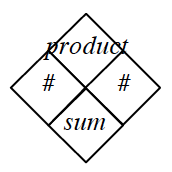1.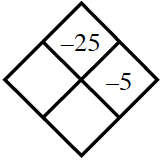Divide $−25$ by $−5$ to find $x$. Then add $x$ and $−5$ to fill the sum box.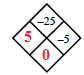Look at the diamond above.

1.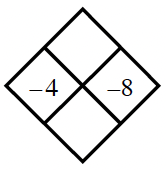Add $−4$ and $−8$ to fill the sumbox, and multiply them to fill the product box.

1.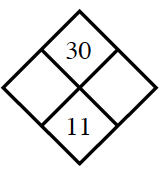What two numbers add to $11$ and multiply to $30$?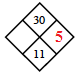You've been given $y$, can you find $x$?

1.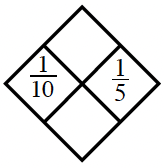This problem is very similar to part (b). Use the skills you've learned about multiplying and adding fractions to complete the diamond.